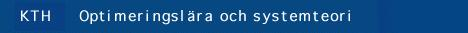## 5B5744 Input/Output Stability and Integral Quadratic Constraints, 3 p, spring 2000

### Examiner and lecturer

Ulf J—nsson (ulfj@math.kth.se), room 3725, Lindstedtsv. 25, phone. 790 84 50.

### Course content

In this course we discuss the operator approach to systems analysis. The emphasis is on the integral quadratic constraints approach to stability analysis. This approach to systems analysis unifies the classical small gain theorem and the passivity theorem in a nice way. We also discuss modeling and analysis of uncertain systems, robust stability and robust performance analysis. The S-procedure lossless theorem is also proved and its use for obtaining necessary conditions for robust stability and performance is illustrated. Another topic is the Kalman-Yakubovich-Popov lemma (KYP) and its use for computation in robust systems analysis. The complete formulation of the KYP lemma is perhaps the single most important result in control theory. The result states the equivalence between a general LQ optimal control problem, a matrix Riccati equation, a condition on the the eigenvalues of an Hamiltonian matrix, a certain frequency domain Linear Matrix Inequality (LMI), and a matrix LMI. Finally, we discuss how the theory can be applied to practical examples using the IQCbeta software.

### Course material

• Lecturenotes [ps].
• System Analysis via Integral Quadratic Constraints by A. Megretski and A. Rantzer.
IEEE Transactions on Automatic Control, 42(6), 1997.